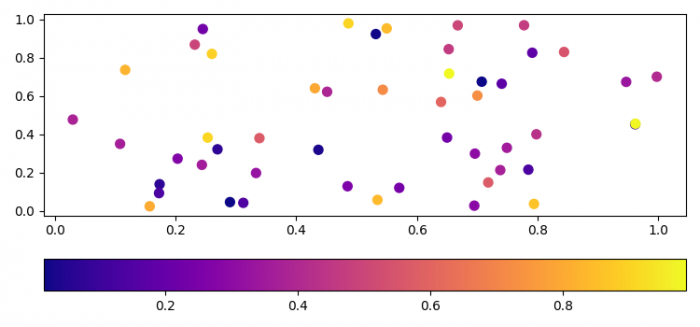# How to make colorbar orientation horizontal in Python using Matplotlib?

To make colorbar orientation horizontal in Python, we can use orientation="horizontal" in the argument.

## Steps

• Set the figure size and adjust the padding between and around the subplots.
• Create random x, y and z data points using numpy.
• Create a figure and a set of subplots.
• Use scatter() method to plot x, y and z data points.
• Create a colorbar for a ScalarMappable instance, with horizontal orientation.
• To display the figure, use show() method.

## Example

import numpy as np
import matplotlib.pyplot as plt

plt.rcParams["figure.figsize"] = [7.50, 3.50]
plt.rcParams["figure.autolayout"] = True

x, y, z = np.random.rand(3, 50)
f, ax = plt.subplots()

points = ax.scatter(x, y, c=z, s=50, cmap="plasma")
f.colorbar(points, orientation="horizontal")

plt.show()

## Output